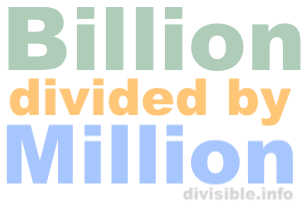Billion divided by Million CalculatorWelcome to our Billion divided by Million Calculator. When dealing with billions and millions, we are dealing with a lot of zeros.

When entering all of those zeros, it is easy to make a mistake, and many calculators may not even allow you to enter that many zeros or digits.

Use our Billion divided by Million Calculator to easily divide billions by millions! Please enter your math problem below so we can solve it for you.

billion divided by  million

Here are some examples of what our Billion divided by Million Calculator can calculate for you.

What is 1 billion divided by 1 million?

What is 7 billion divided by 1 million?

What is 1 billion divided by 10 million?

What is 1 billion divided by 100 million?

What is 50 billion divided by 350 million?

What is 4 billion divided by 2 million?

What is 13 billion divided by 5 million?

What is 6 billion divided by 10 million?